ROV05_6.MWS

Part II. Curves with MAPLE

Chapter 5. Plane Curves in Rectangular Coordinates

Rovenski Vladimir, Haifa

> restart:

5.2 Plotting of Cycloidal Curves

Examples .

1. Plot in the interval t in [-3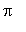, 3] the cycloid for h=1 and its trochoids : prolate for h>1 and curtate for 0<h<1.

> p:=array(-1..1): for i from -1 to 1 do h:= 1+i*8/10: p[i]:=plot([t-h*sin(t), 1-h*cos(t), t=0..5*Pi], title=convert(H=h, string)) od:

> plots[display](p, tickmarks=[3,2], thickness=2, scaling=constrained);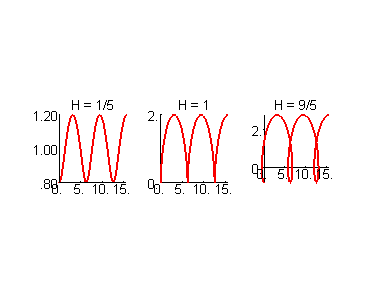> plots[display]([seq(p[i], i=-1..1)], scaling=constrained);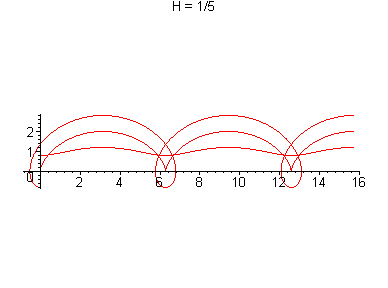>

2. Plot the tables of cycloidal curves with changing parameters with the modulus m= a/b.

For an epicycloid the program is the following:

> p:=array(1..3, 3..5): h:=1:

> for a from 1 to 3 do for b from 3 to 5 do p[a,b]:=plot([[(1+a/b)*cos(a/b*t)-h*a/b*cos(t+a/b*t),(1+a/b)*sin(a/b*t)-h*a/b*sin(t+a/b*t), t=0..2*b*Pi],[cos(t), sin(t), t=0..2*Pi]], thickness=[2, 1], linestyle=[1, 2], title=convert(a/b, string)) od od:

> plots[display](p, axes=none, scaling=constrained);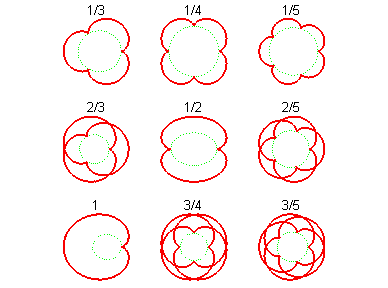>

For modeling hypocycloids we put p:=array(-3..-1, 3..5) in the above program and use the cycle

for a from -3 to -1 do for b from 6 to 8 do .

For modeling prolate and curtate trochoids assume h:=1.5 and then h:=0.5 .

5.3 Experiment With Polar Coordinates

Experiment up and down, around and near [Kos] for some other values k= 6, 7, ... or another relationship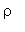= f(t).

> p:=array(1..4, 1..10): # p:=array(1..4, 1..10):

> for a from 1 to 4 do for b from 1 to 10 do p[a,b]:=plot([[sin(5*t)*cos(a*t), sin(5*t)*sin(b*t), t=0..2*Pi], [cos(t),sin(t), t=0..2*Pi]], thickness=[2,1], linestyle=[1,2], title=convert([a,b], string)) od od:

> plots[display](p, scaling=constrained, axes=none);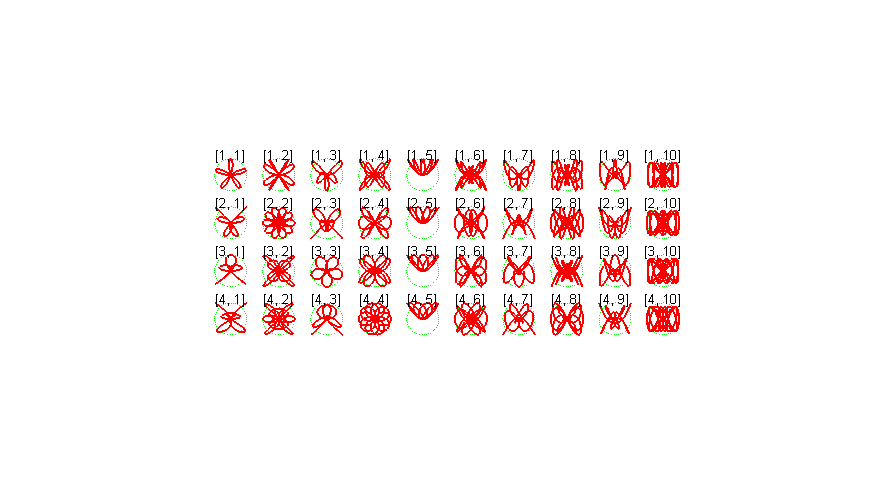>

5.4 Some Other Remarkable Curves

1. The Lissajous curve r(t)= [a sin(nt+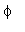), b sin(t)]

> n:=2: phi:=0: a:=1: b:=1: # enter your data

> plot([a*sin(n*t+phi), b*sin(t), t=0..4*Pi]);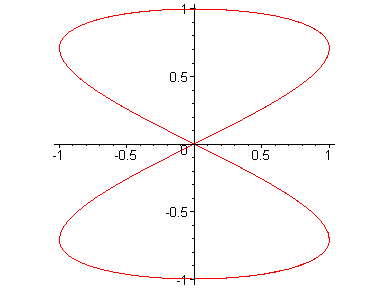>

2. The clothoid

> p:=[int(cos(s*s/2), s=0..t), int(sin(s*s/2), s=0..t)];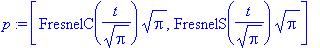> plot([p, p, t=-7..7], scaling=constrained);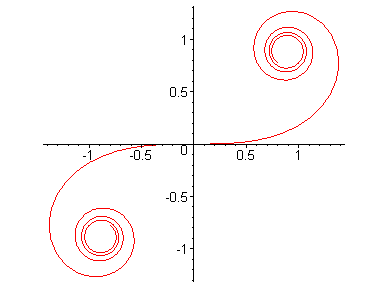>

3. The patterned curves (in mechanics these curves are called elastics ). We plot them when a=/2,, 2.

> a:=2*Pi: p:=[int(cos(a*sin(s)), s=0..t), int(sin(a*sin(s)), s=0..t)]:

> plot([p, p, t=-2*Pi..2*Pi], scaling=constrained);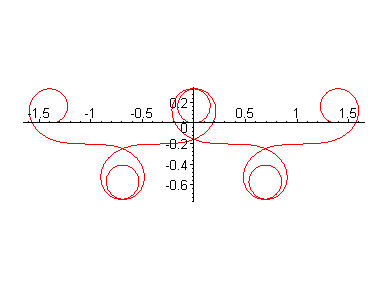4. The SICI spiral r (t) =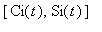, where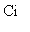is the integral cosine andis the integral sine.

> plot([Ci(t), Si(t), t=1.5..30], axes=framed);>

5.5 Level Curves, Vector Fields, and Trajectories

An implicitly given curve f(x,y)= 0 can be plotted using the command implicitplot from the library plots .

> plots[implicitplot](x^3+y^3-3*x*y, x=-2..2, y=-2..2, grid=[40,40], tickmarks=[3,3]); # folium of Descartes.>

Using the functionone can plot the disconnected curve (perturbed folium of Descartes).

> plots[implicitplot](x^3+y^3-3*x*y+.1, x=-2..2, y=-2..2, grid=[40,40], tickmarks=[3,3]);Implicitly given curves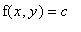for some values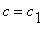, ...,.

> plots[contourplot](sin(x*y), x=-Pi..Pi, y=-Pi..Pi, grid=[50,50], contours=[-0.8, -1/2, 0, 1/2, 0.8]);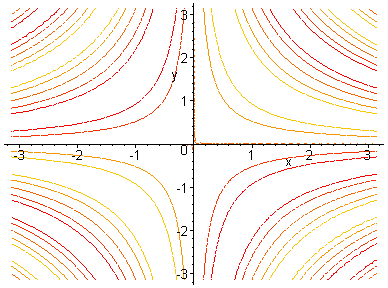The graph of the density of level curves of the function

> plots[densityplot](sin(x*y), x=-Pi..Pi, y=-Pi..Pi, grid=[30,30], axes=boxed);The graph of the gradient vector field for the above function in two variables

> plots[gradplot](sin(x*y), x=-Pi..Pi, y=-Pi..Pi, arrows=SLIM);The graph of an arbitrary two-dimensional vector field

> plots[fieldplot]([y*cos(x), x*cos(y)], x=-Pi..Pi, y=-Pi..Pi, arrows=SLIM);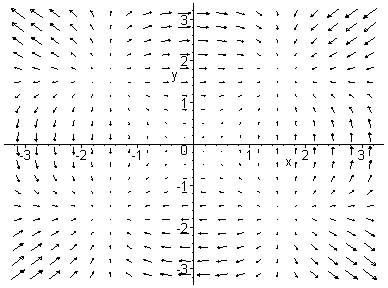The solution (curve) of ODEs.

a)

> DEtools[DEplot](diff(y(x),x,x,x)+x*sqrt(abs(diff(y(x),x)))+x^2*y(x), {y(x)}, x=-4..5, [[y(0)=0, D(y)(0)=1, (D@@2)(y)(0)=1]], stepsize=.1);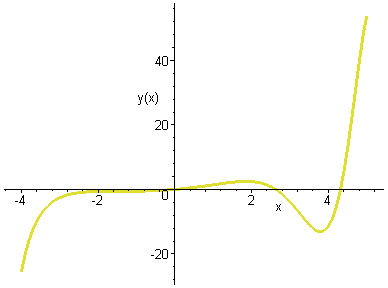>

b)

> f1:= diff(y(x), x, x, x)+x*sqrt(abs(diff(y(x), x)))+x^2*y(x); F1:=dsolve({f1, y(0)=0, D(y)(0)=1, D(D(y))(0)=1}, y(x),type=numeric); plots[odeplot](F1,[x,y(x)],-4..5);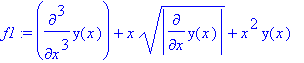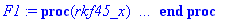Two integral curves and also the direction field for the system of ODEs.

> DEtools[DEplot]({diff(x(t),t)=x(t)*(1-y(t)), diff(y(t),t)=.3*y(t)*(x(t)-1)}, [x(t),y(t)], t=-7..7, [[x(0)=1.2,y(0)=1.2], [x(0)=1,y(0)=.7]], stepsize=.2, title=`Lotka-Volterra model`, arrows=MEDIUM, method=rkf45);The trajectory of the system of three ODEs in the plane.

> DEtools[DEplot]({D(x)(t)=y(t)-z(t), D(y)(t)=z(t)-x(t), D(z)(t)=x(t)-2*y(t)}, {x(t),y(t),z(t)},t=-2..2, [[x(0)=1, y(0)=0, z(0)=2]], stepsize=.05, scene=[z(t),x(t)], arrows=MEDIUM, method=classical[foreuler]);Examples .

1. How to use the library plottools .

> restart: with(plottools): disk([-0.4,0.4],0.1, color=blue): head:=ellipse([0,0.5], 0.7, 0.9, color=black): mouth:=arc([0,0.1], 0.35, 5/4*Pi..7/4*Pi, color=red,thickness=7): eyes1:=disk([0.4,0.4],0.1, color=blue): eyes2:=disk([-0.4,0.4],0.1, color=blue): nose:=line([0,0.35], [0,-0.1], color=black, thickness=5):

> plots[display]({head, eyes1, eyes2,nose, mouth}, scaling=constrained, axes=none); # happy face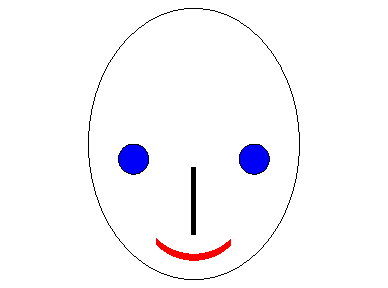>

2. Write the analogous program using the library geometry .

5.6 Level Curves of Functions and Extremal Problems

1. Experimental approach.

> restart: with(plots): f:=sqrt(x^2+y^2): g:=sqrt((x-d)^2+y^2):

```Warning, existing definition for changecoords has been overwritten
```

> G:=simplify((f^2+g^2-d^2)/(2*f*g)); P:=i->implicitplot(subs(d=1, G)=i/10, x=-3..3, y=-3..3, grid=[70,70]):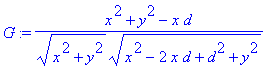> display([seq(P(i), i=0..10)], scaling=constrained);>

2. Geometrical approach

Hints . We find the optimal anglefrom the equation tan()= d/{2|y|}.

> f1:=sqrt(y^2+d^2/4): f2:=(b*y-1)/sqrt(a^2+b^2):

> solve(f1^2=f2^2, y);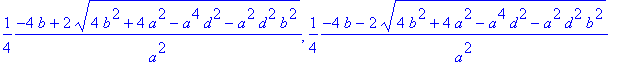3. Analytical approach :

Method 3a .

> f:=sqrt(t^2+((1-b*t)/a)^2): g:=sqrt(t^2+(d-(1-b*t)/a)^2): h:=d: F:=simplify((f^2+g^2-h^2)/(2*f*g));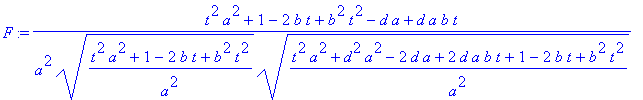> dF:=simplify(diff(F, t));> T:=[solve(numer(dF)=0, t)]; P:=simplify([(b*T-1)/a, T]);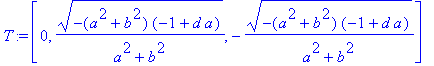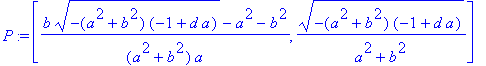>

Method 3b .

> restart: f:=sqrt(x^2+y^2): g:=sqrt((x-d)^2+y^2): F:=a*x+b*y-1:

> G:=simplify((f^2+g^2-d^2)/(2*f*g));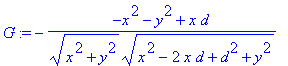> with(linalg): GradG:=grad(G, [x,y]): GradF:=grad(F, [x,y]):

```Warning, protected name norm has been changed and unprotected
```

```Warning, protected name trace has been changed and unprotected
```

> s:=[solve({F, seq(GradG[i]=lambda*GradF[i], i=1..2)},{x, y, lambda})];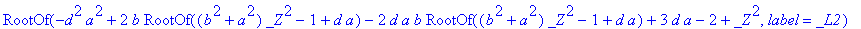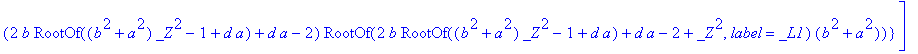> x0:=allvalues(-(b*RootOf((b^2+a^2)*_Z^2-1+da)-1)/a); y0:=allvalues(RootOf((b^2+a^2)*_Z^2-1+da));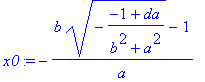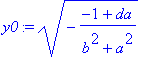> G0:=subs({x=x0, y=y0}, G);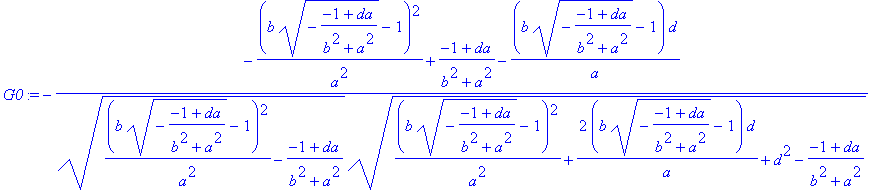>

Conclusion : The point of the best view with y>0 has coordinates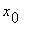= (b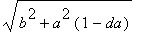/(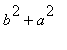)-1)/a,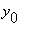=/().